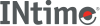﻿ pdd (SDM)INtime SDK Help
pdd (SDM)

Displays the page directory, which is a table containing entries about the secondary page tables. For the meaning of each entry in the display, see the description of the `.component` parameter.

Paging information in the user's manual or programmer's reference for your microprocessor.

```[count] pdd [(index) [.component]]
```

#### Parameters

`count`
A decimal number specifying how many entries from the page directory to display. If you don't specify `count` or `(index)`, the default is to display a screenful of entries until you type `Q` to quit. You can increment the display one screen at a time by typing <Space>, or one line at a time by typing <CR>. If you don't specify `count` but do specify `(index)`, the default value for `count` is 1.
`(index)`
The index into the directory to begin the display. For example, to display 5 entries beginning with entry 11 (index 10, since the index of page tables begins with 0), enter:
```5pdd(10t)
```
`.component`
To display only a single component of a specific directory entry, after the `(index)` enter a period (.) followed by one of these component abbreviations:
`ADDR`
Base address of the second-level page table.
`AV`
Available bits for use by the OS.
`S`
(Pentium† processor-specific) Size bit: 0 means 4 KB page.
`A`
Accessed bit: 1 means read or write to page table.
`PCD`
Page-level Cache Disable bit: 1 means caching is disabled.
`PWT`
Page-level WriteThrough bit: 1 is writethrough, 0 is writeback.
`U`
User/Supervisor bit: 1 is application code and data, 0 is OS memory.
`W`
`P`
Present bit: 1 means the page table is present in memory.

#### Examples

1. The following command displays entries about the first six page tables. See the description of the component parameter above for the meaning of each field. The fifth entry (index 4) is not present in memory.
```..6pdd(0)
PDIR(0T) ADDR=00273000 AV=0 S=0 A=1 PCD=0 PWT=0 U=1 W=1 P=1
PDIR(1T) ADDR=00274000 AV=0 S=0 A=1 PCD=0 PWT=0 U=1 W=1 P=1
PDIR(2T) ADDR=00275000 AV=0 S=0 A=1 PCD=0 PWT=0 U=1 W=1 P=1
PDIR(3T) ADDR=00276000 AV=0 S=0 A=1 PCD=0 PWT=0 U=1 W=1 P=1
PDIR(4T) ffc01ffe         Not Present
PDIR(5T) ADDR=006c1000 AV=0 S=0 A=1 PCD=0 PWT=0 U=1 W=1 P=1
```
2. The following command displays just the base address of the fourth page table.
```..pdd(3).addr